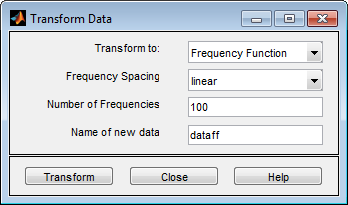Transform Time-Domain Data in the App

In the System Identification app, time-domain data has an icon with a white background. You can transform time-domain data to frequency-domain or frequency-response data. The frequency values of the resulting frequency vector range from 0 to the Nyquist frequency ${f}_{S}=\pi }{Ts}$, where Ts is the sample time.

Transforming from time-domain to frequency-response data is equivalent to estimating a model from the data using the spafdr method.

1. In the System Identification app, drag the icon of the data you want to transform to the Working Data rectangle.

2. In the Operations area, select <--Preprocess > Transform data in the drop-down menu to open the Transform Data dialog box.

3. In the Transform to list, select one of the following:

• Frequency Function — Create a new idfrd object using the spafdr method. Go to step 4.• Frequency Domain Data — Create a new iddata object using the fft method. Go to step 6.

4. In the Frequency Spacing list, select the spacing of the frequencies at which the frequency function is estimated:

• linear — Uniform spacing of frequency values between the endpoints.

• logarithmic — Base-10 logarithmic spacing of frequency values between the endpoints.

5. In the Number of Frequencies field, enter the number of frequency values.

6. In the Name of new data field, type the name of the new data set. This name must be unique in the Data Board.

7. Click to add the new data set to the Data Board in the System Identification app.

8. Click to close the Transform Data dialog box.

Related Examples